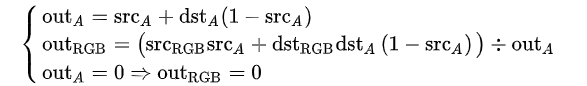# Mix colors () () ratio () : () block

hi, I have been trying (not for homework) to make a mix colors () () ratio () : () block, but I'm not able to. some helpful blocks are in my custom blocks project. can someone please give me the script?

so you mean color blending?i think DST is the color behind and SRC is the color above. and the alpha value should be a fraction between 0 and 1. I think if you want it to be an integer between 0 and 255, then you would need to replace (1 - src_a) with (255 - src_a). That might work. Edit: Wait no try using (1 - (src_a / 255)) Hyperblocks would be useful in calculating this.

Oh well you might have modify that equation a little bit to account for the ratio factor.

Here is what I got:

a and b are three-item lists and p is a number between 0 and 100.

To show that it actually works I picked two thumbnails of Scratch projects I made (because I knew they would be the same width and height) (actually one of the projects has been deleted for a very long time) and blended their colors together and it works see look

just divide the first number by the second nummber

okay. can you make the lists support alpha (transparency 1-100)?

uhh i'm not sure how that'd work

umm... ok. does anyone else know how to add alpha support to pumpkinhead's script?

shouldn't you try to figure it out yourself while waiting? by somehow combining the equation that combines two alpha images in my first reply, and the equation that blends two RGB colors by a value i figured out in the script i gave you?

in the script i gave you it's the outRGB equation i gave you except i replaced srcA with (1 - p), dstA with (p), and removed (1 - srcA)

ok after some testing i found out it's just the equation i gave you but you simply have to multiply the mix factor by src's alpha

where is the [alpha %] of rgba () block?

i made that block, it should be straightforward to make. that just reports the alpha of the color between 0 and 1

ok, i'll try it.

how do you get the input to be a color, using hacks?

the color block is in my custom blocks project. the spec is %clr

It's kind of weird to use the two colors' original alpha values when doing a blend, imho. You should ignore their alphas and just set them to ratio and 1-ratio or something.

huh?

You're trying to set the blending proportion explicitly based on inputs to your block. Alpha (the A in RGBA) does exactly that -- determine how much this RGBA value contributes to a blend. So you're combining two different blend settings: the one in your block's inputs and the one in the pixels. I would interpret your block's semantics as "never mind how the pixels think they should be blended; blend them this way instead."

I don't understand.